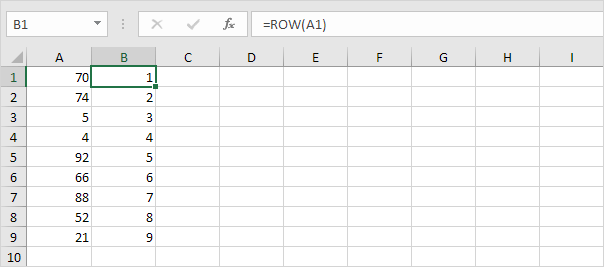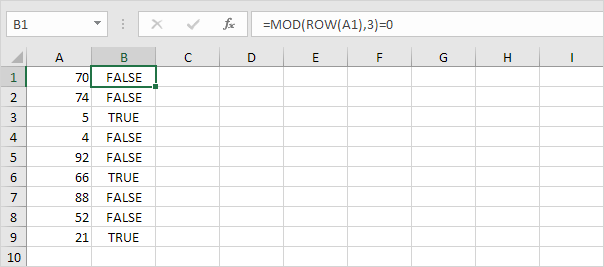# Sum Every nth Row

This example shows you how to create an array formula that sums every nth row in Excel. We will show it for n = 3, but you can do this for any number.

1. The ROW function returns the row number of a cell.2. The MOD function gives the remainder of a division. For example, for the first row, MOD(1,3) = 1 because 1 divided by 3 equals 0 with a remainder 1. For the third row, MOD(3,3) = 0 because 3 divided by 3 equals 1 with a remainder of 0. As a result, the formula returns 0 for every 3rd row.Note: change the 3 to 4 to sum every 4th row, to 5 to sum every 5th row, etc.

3. Slightly change the formula as shown below.4. To get the sum of the product of these two ranges (FALSE=0, TRUE=1), use the SUM function and finish by pressing CTRL + SHIFT + ENTER.Note: The formula bar indicates that this is an array formula by enclosing it in curly braces {}. Do not type these yourself. They will disappear when you edit the
formula.

Explanation: The product of these two ranges (array constant) is stored in Excel’s memory, not in a range. The array constant looks as
follows.

{0;0;5;0;0;66;0;0;21}

This array constant is used as an argument for the SUM function, giving a result of 92.

Previous articleShade Alternate Rows in Excel
Next articleTax Rates in Excel